Frequency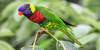the number of plots, stations, counts (visits), or intervals in which a species is detected; when expressed as a fraction of the total sampled, it becomes relative frequency (Ralph 1981:578) (cf Density).
Found on http://www.encyclo.co.uk/local/22030

frequencyA property of a wave that describes how many wave patterns or cycles pass by in a period of time. Frequency is often measured in Hertz (Hz), where a wave with a frequency of 1 Hz will pass by at 1 cycle per second.
Found on http://www.encyclo.co.uk/local/20040

FrequencyThe number of repetitions per unit time of the oscillations of an electromagnetic wave (or other wave). The higher the frequency, the greater the energy of the radiation and the smaller the wavelength. Frequency is measured in Hertz.
Found on https://www.encyclo.co.uk/local/20087

FrequencyThe number of times an event or an item occurs.
Found on http://www.encyclo.co.uk/local/20140

frequency[Noun] How often something happens. Waves used to transmit signals, for example, television or radio.
Example: The frequency of the bus service has been cut from four a day to just two.
Found on http://www.bbc.co.uk/skillswise/glossary/

FrequencyThe number of complete cycles of a periodic waveform that occur in a one second period. Usually specified in Hertz (formerly cycles per second).
Found on http://www.zoo.co.uk/~z0001325/Glossary.html

frequency[n] - the number of occurrences within a given time period (usually 1 second) 2. [n] - the ratio of the number of observations in a statistical category to the total number of observations 3. [n] - the number of observations in a given statistical category
Found on http://www.webdictionary.co.uk/definition.php?query=frequency

Frequency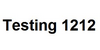Practically speaking, high frequency means high pitch and low frequency means low pitch.
Found on http://www.encyclo.co.uk/local/20447

FrequencyThe number of cycles of a waveform occurring in a second.
Found on http://www.encyclo.co.uk/local/20447

Frequency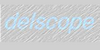The number of waves passing a point in a given time, usually 1 second. Frequency is measured in hertz and is equal to the speed of the wave divided by their wavelength. Longer wavelengths have lower frequency and vice-versa.
Found on http://www.encyclo.co.uk/local/20448

Frequency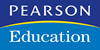the average number of times that a member of the target audience will have been exposed to an advertisement during a specified period.
Found on https://www.encyclo.co.uk/local/20463

Frequency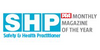The number of complete cycles of an electromagnetic wave in a second. Unit: Hertz, symbol: Hz. 1 Hz = 1 cycle per second.
Found on http://www.encyclo.co.uk/local/20474

frequency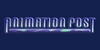in sound: how high or low a musical note is. This is directly analogous to colour in the visual realm, where different colours are due to their frequency value in the electromagnetic spectrum
Found on http://www.animationpost.co.uk/doping/glossary.htm

Frequency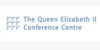The rate of vibration of an electrical or mechanical oscillation. Measured by the number of complete cycles executed in 1 second. In audio terminology, frequency range is considered to be from 20 cycles per second, or 20 Hertz (Hz), up to 20,000 cycles per second, or 20 kilohertz.
Found on http://www.encyclo.co.uk/local/20523

Frequency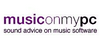The rate per second at which an oscillating body vibrates. Usually measured in Hertz (Hz), humans can hear sounds with frequencies in the range of 20 Hz to 20 kHz
Found on http://www.encyclo.co.uk/local/20532

Frequency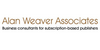The time period between successive issues of a publication such as weekly, monthly or quarterly
Found on http://www.encyclo.co.uk/local/20553

Frequency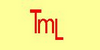the rate per second at which an oscillating body vibrates. Usually measured in Hertz (Hz), humans can hear sounds whose frequencies are in the range 20 Hz to 20kHz. In audio, the number of repeating cycles of change in air pressure or oscillations in voltage, that occur in one unit of time usually a second. Complex sounds are made up of many pure t...

Frequencyâ€` The amount of times that a wave repeats per second, measured in Hertz (which are cycles per second) after Heinrich R. Hertz the man who devised this form of measurement. Or to put it another way, if a soundwave vibrates the air x amount of times a second, this could be said to be it's frequency in Hertz. A young human who hasn't abused their...

Frequency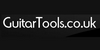the number of cycles per second, which determines pitch (measured in Hertz, Hz)
Found on http://www.encyclo.co.uk/local/20596

Frequency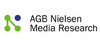The average number of times that members of a target audience who have been counted at least once as viewers to a schedule of advertising spots (or sequence of programmes), have counted as viewers.
Found on http://www.agbnielsen.net/glossary/glossaryQ.asp?type=alpha&jump=none

Frequency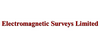The number of complete cycles of an electromagnetic wave in one second. The unit is the Hertz, symbol Hz. Frequency used to be simply called cycles per second. High frequencies are also usually given a prefix, ie: 1000Hz = 1KiloHertz (1KHz) 1000000Hz = 1MegaHertz (1MHz) 1000000000Hz = 1GigaHertz (1GHz) 1000000000000Hz = 1TeraHertz (1THz)
Found on http://www.em-surveys.co.uk/Definitions%201.html

Frequency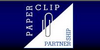Frequency is the average number of times your target audience is exposed to your message. People consume more than one type of media so frequency calculations take this into account. For example, someone may read the Financial Times and Marie Claire and also watch television. â€˜This many people saw my message this many times. For example, 20% ...
Found on http://www.paperclippartnership.co.uk/public-relations-definitions.html

FrequencyThe rate of repetition of periodic motion measured in hertz (cycles per second).Rate of repetition of changes.wheref = frequency [Hz]T = period [s]v = wave speed [ms-1]? = wavelength [m]? = angular frequency [rad s-1]Enter values into 2 of the 5 fields. The others will be calculated.function init(){}For low frequency events (e...
Found on http://www.diracdelta.co.uk/science/source/f/r/frequency/source.html

Frequency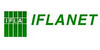The intervals at which a serial is published, e.g., weekly, monthly, bimonthly, quarterly, annually, etc
Found on http://www.ifla.org/VII/s30/pub/mg1.htm#5

Frequency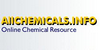The number of repeating corresponding points on a wave that pass a given observation point per unit time.
Found on http://www.encyclo.co.uk/local/20728
No exact match found